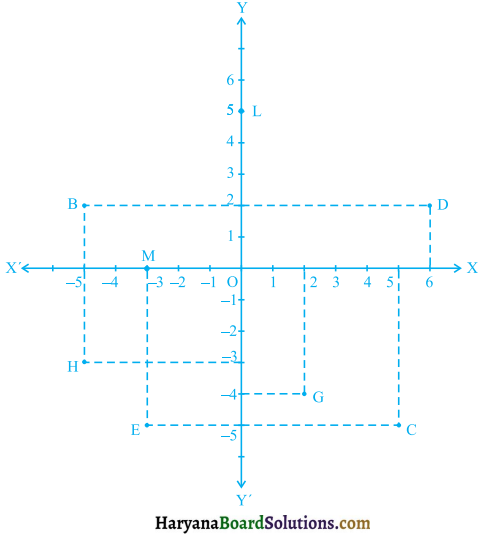# HBSE 9th Class Maths Solutions Chapter 3 Coordinate Geometry Ex 3.2

Haryana State Board HBSE 9th Class Maths Solutions Chapter 3 Coordinate Geometry Ex 3.2 Textbook Exercise Questions and Answers.

## Haryana Board 9th Class Maths Solutions Chapter 3 Coordinate Geometry Exercise 3.2

Question 1.
Write the answer of each of the following questions :
(i) What is the name of horizontal and vertical lines drawn to determine the position of any point in the cartesiar plane?
(ii) What is the name of each part of the plane formed by these two lines?
(iii) Write the name of the point where these two lines intersect.
Solution :
(i) The name of the horizontal line is z-axis and vertical line is y-axis.
(ii) Each part of the plane formed by these two lines is known as quadrant.
(iii) Name of the point where these two lines intersect is origin. It is denoted by O.Question 2.
See figure 3.10, and write the following:(i) The coordinates of B.
(ii) The coordinates of C.
(iii) The point identified by the coordinates (-3, -5).
(iv) The point identified by the coordinates (2, – 4).
(v) The abscissa of the point D.
(vi) The ordinate of the point H.
(vii) The coordinates of the point L.
(viii) The coordinates of the point M.
Solution :
(i) Distance of the point B from the y-axis is – 5 units. Therefore, the x-coordinate or abscissa of the point Bis – 5. The distance of the point B from the x-axis is 2 units. Therefore, theycoordinate i.e., the ordinate of the point B is 2. Hence, the coordinates of the point B are (-5, 2).

(ii) Distance of the point from the y-axis is 5 units and that of from x-axis is 5 units. Hence, the coordinates of point Care (5, – 5).

(iii) The point identified by the coordinates (-3, -5) is E.

(iv) The point identified by the coordinates (2, – 4) is G.

(v) Distance of the point D from the y-axis is 6 units, so the x-coordinate i.e., the abscissa of the point D is 6.

(vi) Distance of the point H from x-axis is – 3 units. So, the y-coordinate i.e., the ordinate of the point H is – 3.

(vii) The distance of the point L from y-axis is 0 unit and that of from x-axis is 5 units. Hence, the coordinates of the point L are (0, 5).

(viii) The distance of the point M from y-axis is – 3 units and that of from x-axis is 0 unit. Hence, the coordinates of the point Mare (-3, 0).